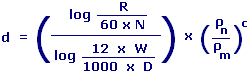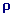d-Exponent Calculator Enter value and click on calculate. Result will be displayed.R = Penetration Rate N = Rotary Speed W = Weight on Bit D = Drill Bit Diametern = Mud Weight Equivalentm = Mud Weight Used C = Shale Compactibility Coefficient
 Enter your values: Penetration Rate: Ft / Hr Rotary Speed: Rev / Minute Weight on Bit: 1000 Lb Drill Bit Diameter: Inches Mud Weight Equivalent: Lb / Gal Mud Weight Used: Lb / Gal Shale Compactibility Coefficient: Result: d-Exponent:

 Web www.calculatoredge.com
 This concept was first developed by Jorden & Shirley This is a factor for evaluating drilling rate and predicting abnormal pore pressure zones.

All things being equal, the d-exponent should increase with depth when drilling in a normal pressure section. A reversal of this trend is an indication of drilling into potential overpressures.LINKSDISCLAIMERCONTACT US